Forum of Mathematics, Sigma ( IF 1.464 ) Pub Date : 2020-11-20 , DOI: 10.1017/fms.2020.37
José M. Conde-Alonso; Adrián M. González-Pérez; Javier Parcet

Our first result is a noncommutative form of the Jessen-Marcinkiewicz-Zygmund theorem for the maximal limit of multiparametric martingales or ergodic means. It implies bilateral almost uniform convergence (a noncommutative analogue of almost everywhere convergence) with initial data in the expected Orlicz spaces. A key ingredient is the introduction of the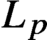$L_p$ -norm of the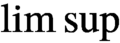$\limsup$ of a sequence of operators as a localized version of a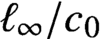$\ell _\infty /c_0$ -valued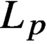$L_p$ -space. In particular, our main result gives a strong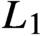$L_1$ -estimate for the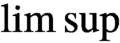$\limsup$ —as opposed to the usual weak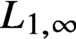$L_{1,\infty }$ -estimate for the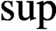$\mathop {\mathrm {sup}}\limits$ —with interesting consequences for the free group algebra.

Let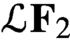$\mathcal{L} \mathbf{F} _2$ denote the free group algebra with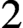$2$ generators, and consider the free Poisson semigroup generated by the usual length function. It is an open problem to determine the largest class inside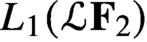$L_1(\mathcal{L} \mathbf{F} _2)$ for which the free Poisson semigroup converges to the initial data. Currently, the best known result is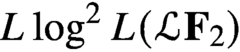$L \log ^2 L(\mathcal{L} \mathbf{F} _2)$ . We improve this result by adding to it the operators in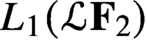$L_1(\mathcal{L} \mathbf{F} _2)$ spanned by words without signs changes. Contrary to other related results in the literature, this set grows exponentially with length. The proof relies on our estimates for the noncommutative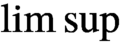$\limsup$ together with new transference techniques.

We also establish a noncommutative form of Córdoba/Feffermann/Guzmán inequality for the strong maximal: more precisely, a weak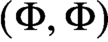$(\Phi ,\Phi )$ inequality—as opposed to weak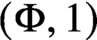$(\Phi ,1)$ —for noncommutative multiparametric martingales and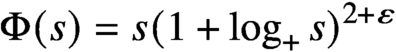$\Phi (s) = s (1 + \log _+ s)^{2 + \varepsilon }$ . This logarithmic power is an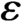$\varepsilon$ -perturbation of the expected optimal one. The proof combines a refinement of Cuculescu’s construction with a quantum probabilistic interpretation of M. de Guzmán’s original argument. The commutative form of our argument gives the simplest known proof of this classical inequality. A few interesting consequences are derived for Cuculescu’s projections.

down
wechat
bug